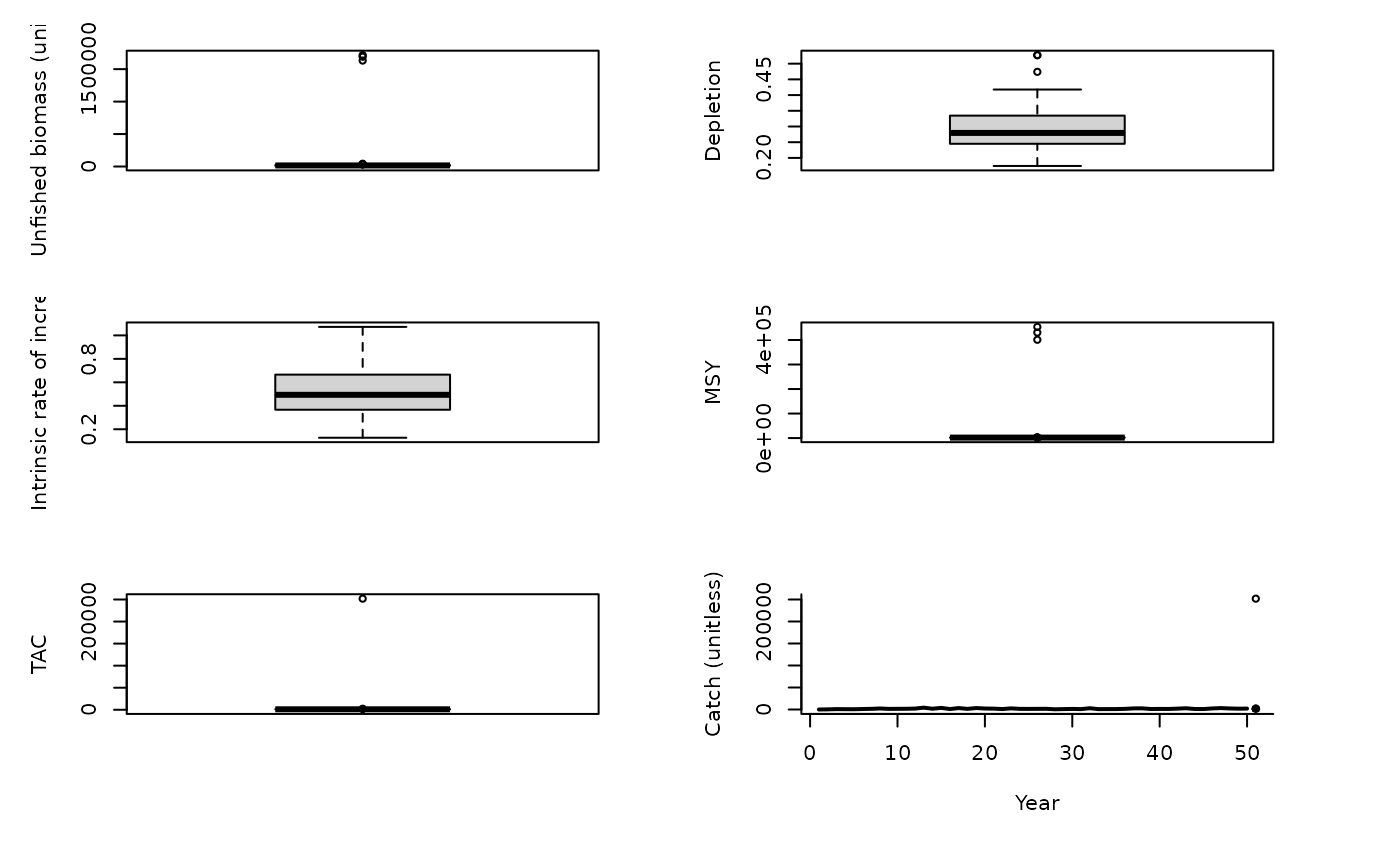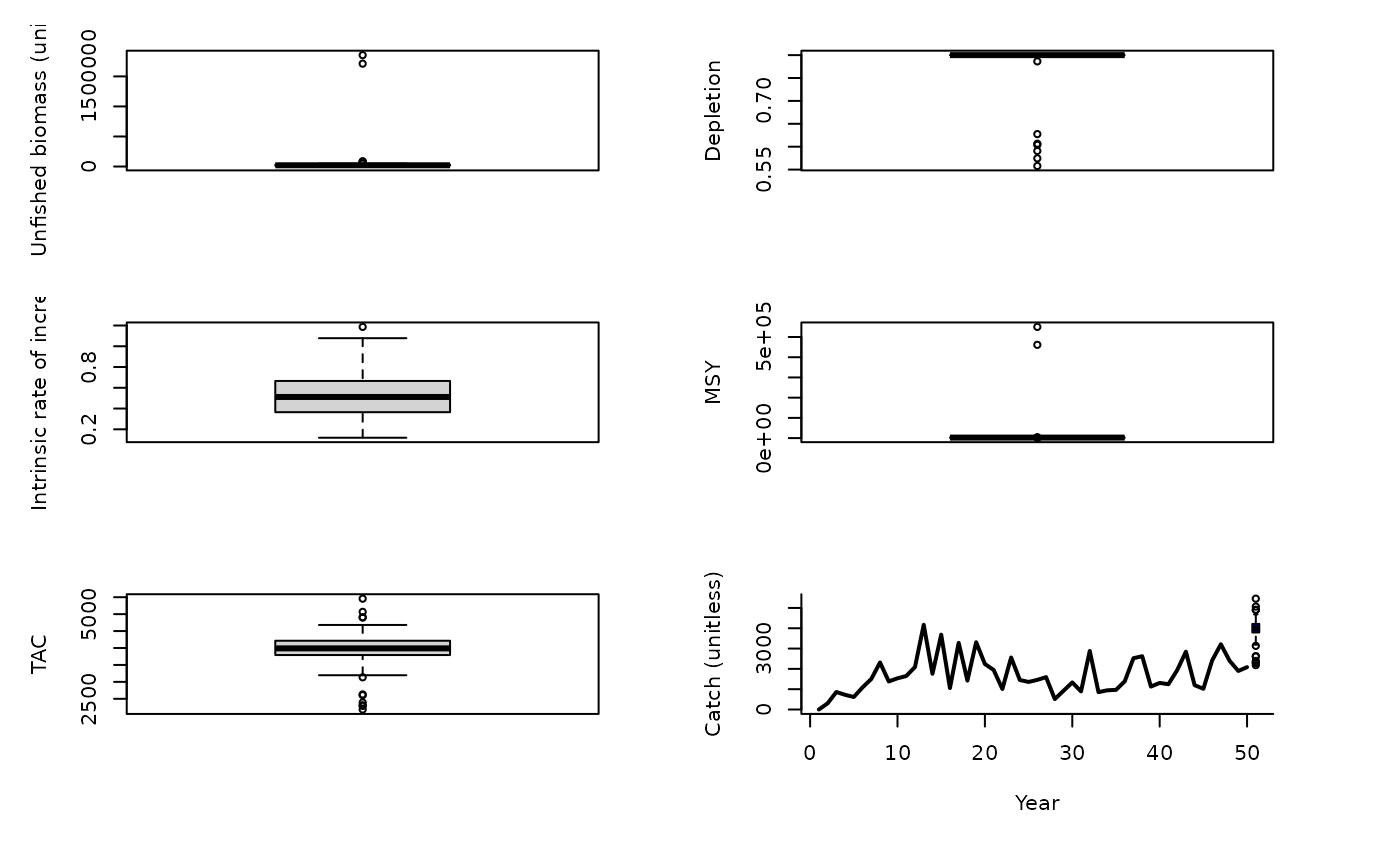A surplus production equivalent of DB-SRA that uses a demographically derived prior for intrinsic rate of increase (McAllister method, below)

SPSRA(x, Data, reps = 100, plot = FALSE)

SPSRA_ML(x, Data, reps = 100, plot = FALSE)

## Arguments

x

A position in the data object

Data

A data object

reps

The number of stochastic samples of the MP recommendation(s)

plot

Logical. Show the plot?

## Value

An object of class Rec-class with the TAC slot populated with a numeric vector of length reps

## Details

The TAC is calculated as: $$\textrm{TAC} = K D \frac{r}{2}$$ where $$K$$ is estimated unfished biomass, $$D$$ is depletion, and $$r$$ is the estimated intrinsic rate of increase.

Like all SRA methods, this MP requires a time-series of catch extending from the beginning of exploitation.

## Functions

• SPSRA: Base version. Requires an estimate of current depletion

• SPSRA_ML: Variant that uses a mean-length mortality estimator to obtain a prior for current stock depletion.

## Required Data

See Data-class for information on the Data object

SPSRA: Cat, Dep, FMSY_M, L50, MaxAge, Mort, steep, vbK, vbLinf, vbt0, wla, wlb

SPSRA_ML: CAL, Cat, Dep, FMSY_M, L50, Lbar, Lc, MaxAge, Mort, Year, steep, vbK, vbLinf, vbt0, wla, wlb

## Rendered Equations

See Online Documentation for correctly rendered equations

Other Surplus production MPs: Fadapt(), Rcontrol(), SPMSY(), SPmod(), SPslope()

T. Carruthers

## Examples

SPSRA(1, MSEtool::SimulatedData, plot=TRUE)#> TAC (median)
#>     1114.265
SPSRA_ML(1, MSEtool::SimulatedData, plot=TRUE)#> TAC (median)
#>     3969.005